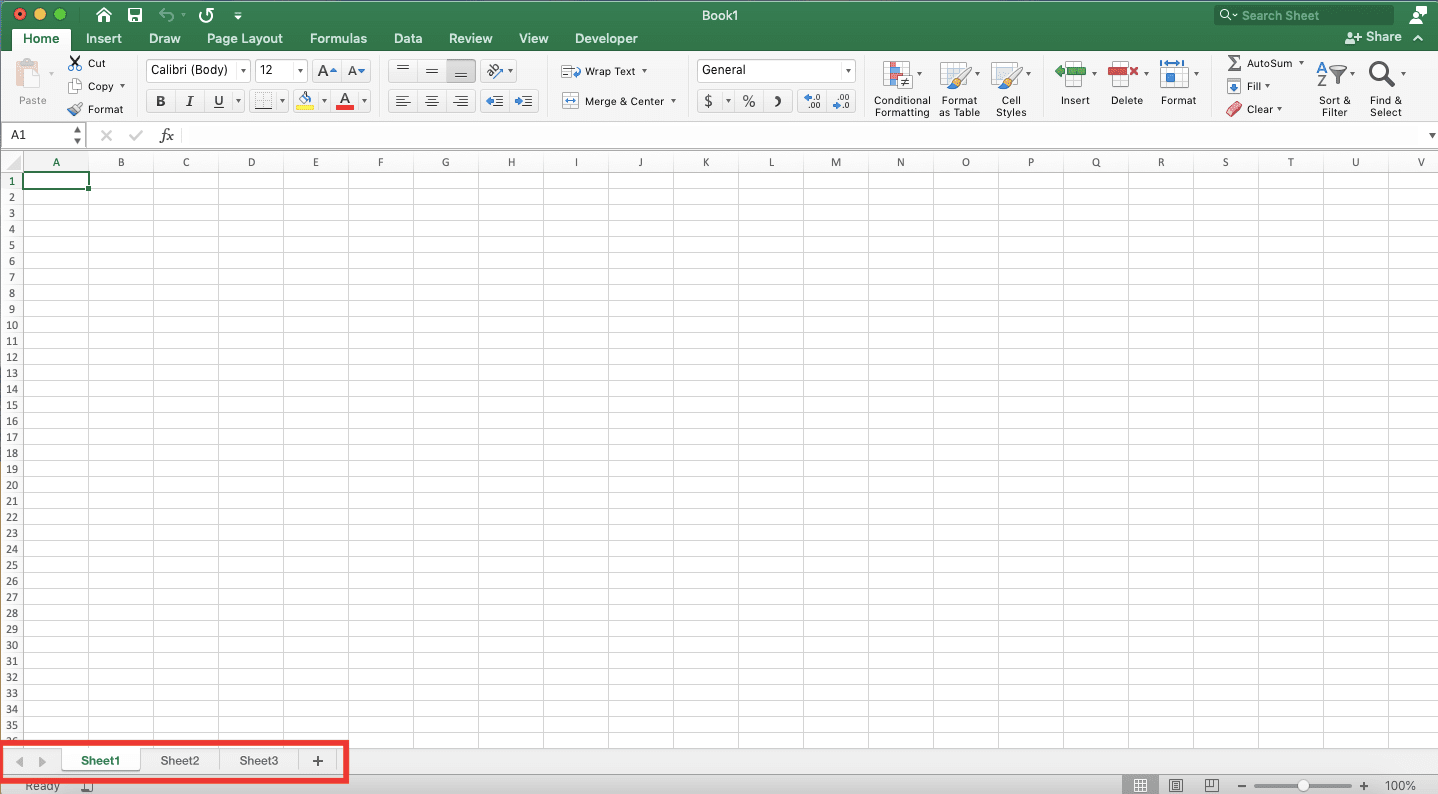Excel Worksheet & Workbook Definition and Difference - Compute Expert

# Excel Worksheet & Workbook Definition and Difference

Home >> Excel Tutorials from Compute Expert >> Excel Tips and Trick >> Excel Worksheet & Workbook Definition and Difference

In this article, we will discuss completely the definition and difference of worksheet and workbook in excel.

A good understanding of the worksheet and workbook is important to have for excel users. After all, they are the place where we do our work in excel. If you haven’t understood these worksheet and workbook phrases, then you will get the complete understanding after reading this article!

Disclaimer: This post may contain affiliate links from which we earn commission from qualifying purchases/actions at no additional cost for you. Learn more

## Worksheet (Sheet) Definition

By definition, a worksheet or sheet is the media we use to input and process our data in excel.

One excel worksheet has many cells we can use to place our data and do our data analysis. We can divide our data per worksheet according to our specific needs.

If needed, we can also interact between worksheets in our data processing. This is usually done if the excel formula we write needs data reference from a different worksheet.

To make your understanding clearer regarding the excel worksheet, you can see the form of one excel worksheet below (in the red box).As you see, what we call a worksheet is the area where you can input and process your data in excel. If you move to another worksheet, then that area shown will be filled with new data. The data depends on the content of the other worksheet.

If you want to move, add, or set other things related to your sheets, you can do it at the bottom. You can see the specific location of it in the screenshot below.Set your worksheets correctly so you can input, process, and separate your data more optimally!

## Workbook Definition

We have discussed the worksheet. But, what about the thing we call a workbook in excel?

Generally, a workbook is a whole part of where you process your data in excel. It can be assumed as a file where all of your worksheets reside.

When you do your work in excel, then the whole thing you see in your excel file is your workbook. The interface of a workbook is what is shown in the screenshot below.In the image, the whole thing in there is what is called your workbook in excel. A workbook also includes various functions available at the top to help you process all the data you have.

## Workbook and Worksheet Difference

Then, what is the difference between workbook and worksheet in excel? If you have understood the explanation given previously, then you might have already known the difference.

In excel, a worksheet is a place where your cells are. It is the location where you put and process your data. If we talk about a workbook, then the scope is larger. It is the excel file where all your worksheets are and it has various menus to help you process your data.

So, if described simply, then a worksheet is a part of a workbook. If we describe a worksheet as one unit in your excel, then a workbook includes all the worksheet units that you have!

Now, have you understood about worksheet, workbook, and their difference? After reading their full explanation above, you should have all you need for that understanding!

Workbook in excel can have many extension types besides xlsx and xls, depending on its content. If you want to know what are those extensions available for an excel workbook, then visit the tutorial here.

Related tutorials you should learn:

Get updated excel info from Compute Expert by registering your email. It's free!